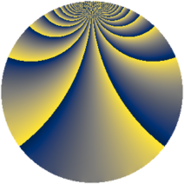# Properties

 Label 1078.2.sLevel $1078$ Weight $2$ Character orbit 1078.s Rep. character $\chi_{1078}(19,\cdot)$ Character field $\Q(\zeta_{30})$ Dimension $320$ Sturm bound $336$

# Related objects

## Defining parameters

 Level: $$N$$ $$=$$ $$1078 = 2 \cdot 7^{2} \cdot 11$$ Weight: $$k$$ $$=$$ $$2$$ Character orbit: $$[\chi]$$ $$=$$ 1078.s (of order $$30$$ and degree $$8$$) Character conductor: $$\operatorname{cond}(\chi)$$ $$=$$ $$77$$ Character field: $$\Q(\zeta_{30})$$ Sturm bound: $$336$$

## Dimensions

The following table gives the dimensions of various subspaces of $$M_{2}(1078, [\chi])$$.

Total New Old
Modular forms 1472 320 1152
Cusp forms 1216 320 896
Eisenstein series 256 0 256

## Trace form

 $$320 q - 40 q^{4} - 12 q^{5} - 64 q^{9} + O(q^{10})$$ $$320 q - 40 q^{4} - 12 q^{5} - 64 q^{9} + 12 q^{11} + 36 q^{15} + 40 q^{16} + 30 q^{17} - 16 q^{22} + 16 q^{23} - 16 q^{25} + 24 q^{26} - 20 q^{29} + 18 q^{31} + 126 q^{33} - 48 q^{36} - 24 q^{37} + 12 q^{38} - 40 q^{39} + 30 q^{40} - 2 q^{44} - 108 q^{45} + 24 q^{47} + 80 q^{51} - 8 q^{53} + 80 q^{57} + 28 q^{58} + 60 q^{59} - 12 q^{60} - 30 q^{61} + 80 q^{64} - 72 q^{66} + 48 q^{67} - 30 q^{68} - 56 q^{71} - 20 q^{72} - 90 q^{73} - 20 q^{74} - 180 q^{75} - 160 q^{78} - 30 q^{79} - 18 q^{80} - 4 q^{81} - 60 q^{82} - 180 q^{85} - 2 q^{86} - 18 q^{88} + 36 q^{89} - 28 q^{92} - 146 q^{93} + 120 q^{94} + 150 q^{95} - 16 q^{99} + O(q^{100})$$

## Decomposition of $$S_{2}^{\mathrm{new}}(1078, [\chi])$$ into newform subspaces

The newforms in this space have not yet been added to the LMFDB.

## Decomposition of $$S_{2}^{\mathrm{old}}(1078, [\chi])$$ into lower level spaces

$$S_{2}^{\mathrm{old}}(1078, [\chi]) \cong$$ $$S_{2}^{\mathrm{new}}(77, [\chi])$$$$^{\oplus 4}$$$$\oplus$$$$S_{2}^{\mathrm{new}}(154, [\chi])$$$$^{\oplus 2}$$$$\oplus$$$$S_{2}^{\mathrm{new}}(539, [\chi])$$$$^{\oplus 2}$$